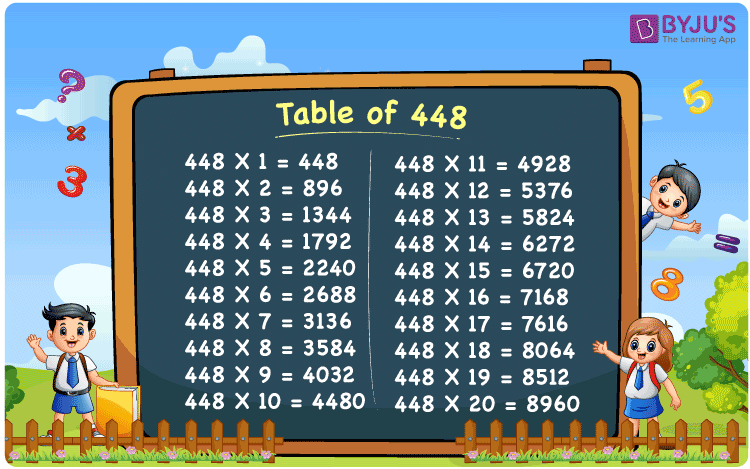Checkout JEE MAINS 2022 Question Paper Analysis : Checkout JEE MAINS 2022 Question Paper Analysis :

# Table of 448

The Multiplication Table for 448 is nothing more than the repetitive addition of the number 448. 448 is an even number that is divisible by 2. 448 is also a multiple of 4. Observe the multiples of 448, they are divisible by 4 as well. You can read more on Divisibility rules.

## Table of 448 Chart## What is the 448 Times Table?

In the table of 448, we are repeatedly adding 448 multiple times. And the table below gives a repetitive addition 10 times for the number 448.

 448 × 1 = 448 448 448 × 2 = 896 448 + 448 = 896 448 × 3 = 1344 448 + 448 + 448 = 1344 448 × 4 = 1792 448 + 448 + 448 + 448 = 1792 448 × 5 = 2240 448 + 448 + 448 + 448 + 448 = 2240 448 × 6 = 2688 448 + 448 + 448 + 448 + 448 + 448 = 2688 448 × 7 = 3136 448 + 448 + 448 + 448 + 448 + 448 + 448 = 3136 448 × 8 = 3584 448 + 448 + 448 + 448 + 448 + 448 + 448 + 448 = 3584 448 × 9 = 4032 448 + 448 + 448 + 448 + 448 + 448 + 448 + 448 + 448 = 4032 448 × 10 = 4480 448 + 448 + 448 + 448 + 448 + 448 + 448 + 448 + 448 + 448 = 4480

## Multiplication Table of 448

Given below is the multiplication table of 448 being done 20 times. The below table helps you with multiplication problems that require the tables of 448.

 448 × 1 = 448 448 × 2 = 896 448 × 3 = 1344 448 × 4 = 1792 448 × 5 = 2240 448 × 6 = 2688 448 × 7 = 3136 448 × 8 = 3584 448 × 9 = 4032 448 × 10 = 4480 448 × 11 = 4928 448 × 12 = 5376 448 × 13 = 5824 448 × 14 = 6272 448 × 15 = 6720 448 × 16 = 7168 448 × 17 = 7616 448 × 18 = 8064 448 × 19 = 8512 448 × 20 = 8960

## Solved Example on Table of 448

Example:

Solve for y:

448 x y = 9000 – 40

Solution: 20

448 x y = 9000 – 40

9000 – 40 = 8960

448 x y = 8960

y = 8960 / 448 = 20.

## Frequently Asked Questions on Table of 448

### Question 1: Is 448 an even number?

Answer: 448 is an even number, integer, natural number but not a prime number.

### Question 2: Is 448 a multiple of 4?

Answer: Yes. 448 is a multiple of 4.